The Vietnamese Mathematical Hobby Group

MATHEMATICAL TEST PAPER #2

Question 1:

(i) Find the second derivative of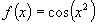(ii) Find a porimitive of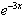(iii) Find the area under the curve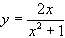between x = 0 and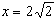.

Question 2:

(i) Prove that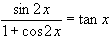(ii) Find the vertex of the parabola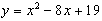(iii) An improperly constructed die has the numbers 1,2,3,4,6 and 6 on its six faces . Given that all faces have equal probability of coming up what is the expected value of the amount shown by the die.

Question 3:

(i) By using one step Newton method, find an expression to that of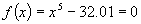which is closer to x = 2.

(ii) The n-th term of a series is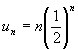. Find the sum of the first ten terms.

(iii) Evaluate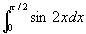Question 4:

(i) Evaluate (a)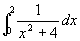(b)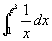(ii) Write down the formula for the expansion of cos(A+B) and deduce that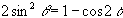.

(iii) An alternating electric current i amperes passing through a given radiator produces heat at the instantaneous rate of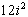calories per second. Find the amount of heat produced in this radiator in one full second by the current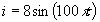where t represents time in seconds. (Hints: you may use the result of part (ii) of the question).

Question 5:

Consider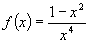,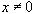(i) Sketch the zeros of this function, i.e. values of x where f(x) = 0.

(ii) Find the turning points of this function, and determine their nature.

(iii) Describe how this function behaves for x = 0.

(iv) Describe how this function behaves for large x.

(v) Give a rough sketch of this function

Question 6:

(i) Expand the expression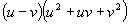(ii) By letting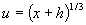and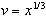or otherwise establish the identity: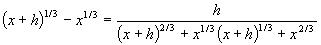(iii) Differentiating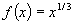from first principle.

Question 7:

(i) Differentiate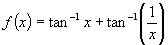.

(ii) By considering a right angled triangle with two sides equal to 1 and x, respectively, or otherwise find the actual value of f(x) for all x > 0.

(iii) By using the fact that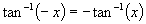or otherwise deduce that the value of f(x) for all x<0.

(iv) Is it possible to assign a value to f(0) so as to make f(x) a function which is continuous at x=0 ? Explain your answer with the aid of a sketch.

Question 8:

(i) If A and B are the roots of the quadratic equation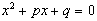, write down the value of (a) A+B (b) AB (c) A2 + B2.

(ii) Let a, b, c and d be the roots of the equation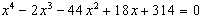(a) Without attempting to locate the individual roots, state the value of their sum S=a+b+c+d.

(b) Similarly state the value of T = ab + ac + ad + bc + bd + cd

(c) From your results for S and T or otherwise find the value of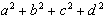.

Question 9:

A man stands on the roof of a tall tower and shoots an arrows ina horizontal direction; the arrow starts 45 m above the ground, with an initial velocity of 30 m/s. You may assume g=10 m/s2.

(i) What time elapses before the arrow strikes the ground.

(ii) How far from the base of the tower does the arrow strike the ground

(iii) What is the accurate angle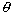betwwen the path of the arrow and the horizontal at the moment the arrow strikes the ground.

(iv) Now assume that there is air friction force in a direction opposite to the velocity of the arrow, and that it is an increasing function of the speed of the arrow. Reason qualitatively, without calculating, to decide whether air friction makes the angleof part (iii) large or smaller; give reasons. (hints: consider the extreme case where air friction is very large, so that it is the dominant force at all but very small speed).

Question 10:

In the 1970s electronic computer were built with valves (rather than transistors). Assume that each particular valve has a probability P=0.00002 of failing during the next hour. (assume that valve failures are independent events, and are independent of the length of past service of the valve)

(i) Find the probability Q that a particular valve operating correctly during the next hour.

(ii) If the computer contains 10,000 valves show that the probability Po of no valve failing during the next hour is 0.82, to two significant figures. (Hints: in the calculation use the first few terms of a binomial expansion rather than logarithm)

(iii) If we are to construct a computer containing 10,000 valves , with a probability of trouble-free operation for one hour at least as high as 0.99, estimate how small must be the probability P of failure of one valve during the next hour.

(iv) With P as in (i) what is the probability of exactly one valve failure during the next hour.